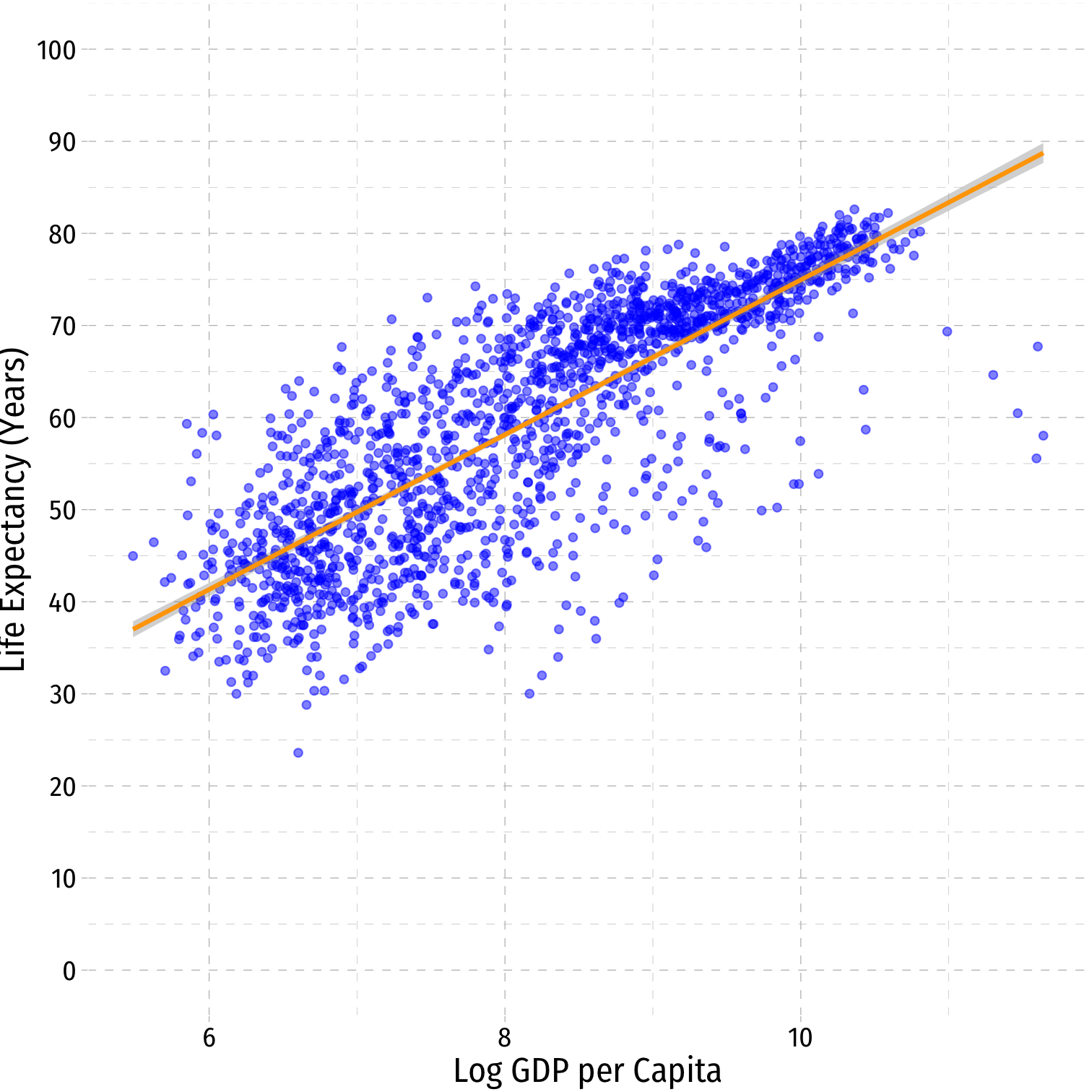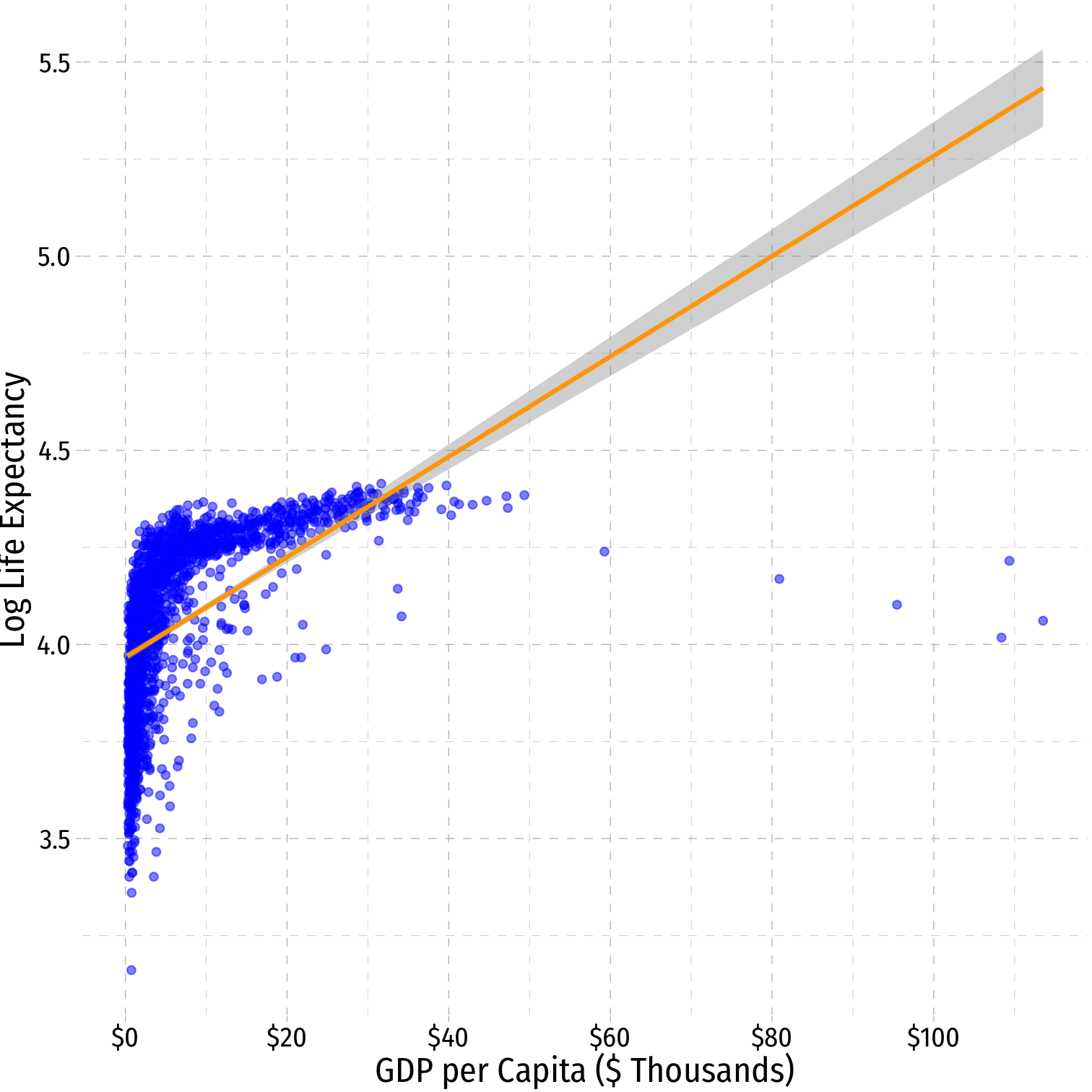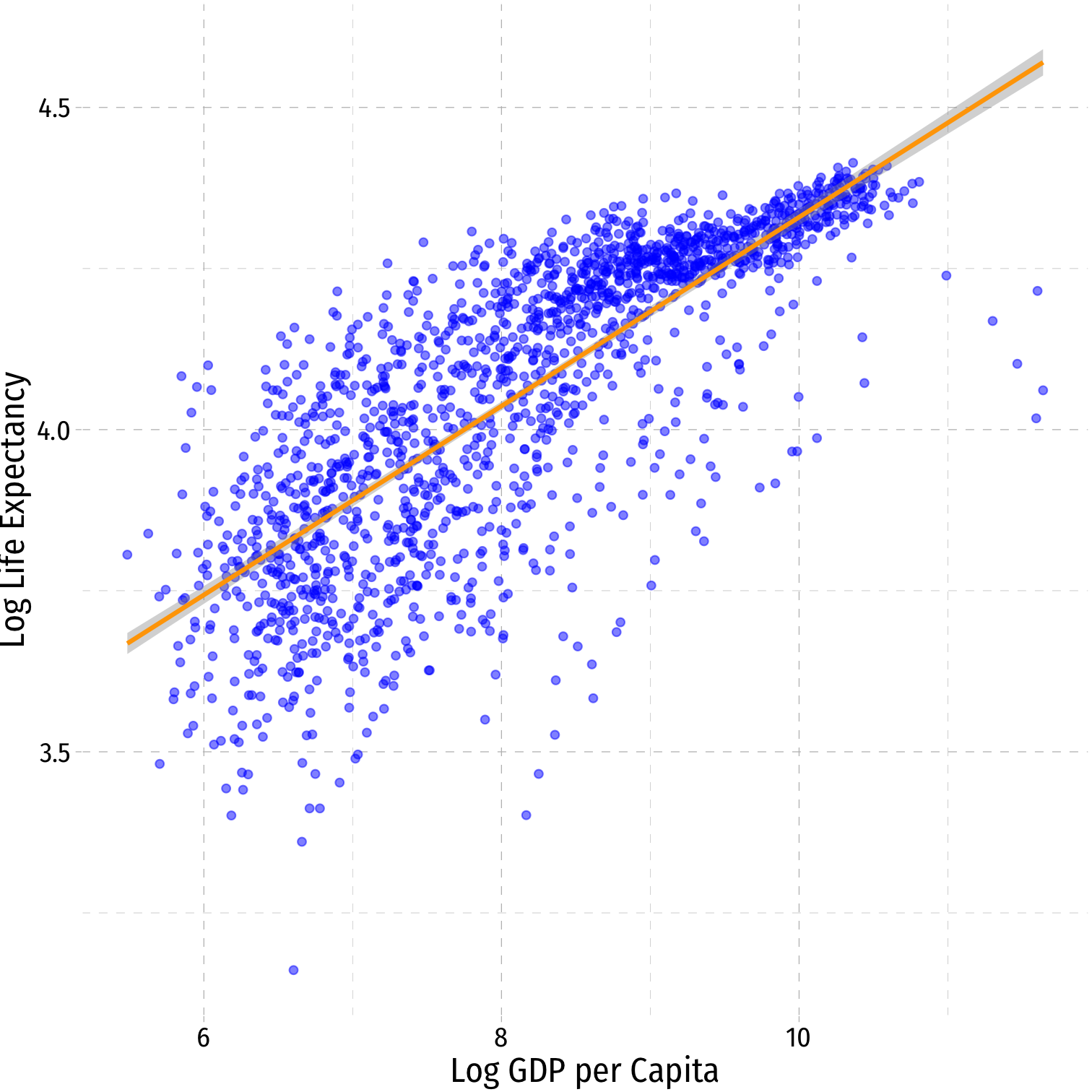# 3.9 — Logarithmic Regression - Class Notes

## Contents

Thursday, November 5, 2020

## Overview

Today, we finish up our view of nonlinear models with logarithmic models, which are more frequently used. We also discuss a few other tests and transformations to wrap up multivariate regression before we turn to panel data: standardizing variables to compare effect sizes, and joint hypothesis tests.

Interpretting logged variables can often be difficult to remember, so here I reproduce the tables that describe the interpretations of the marginal effect of $$X \rightarrow Y$$, as well as some visual examples from the slides:

Model Equation Interpretation
Linear-Log $$Y=\beta_0+\beta_1 \mathbf{ln(X)}$$ 1% change in $$X \rightarrow \frac{\hat{\beta_1}}{100}$$ unit change in $$Y$$
Log-Linear $$\mathbf{ln(Y)}=\beta_0+\beta_1X$$ 1 unit change in $$X \rightarrow \hat{\beta_1}\times 100$$% change in $$Y$$
Log-Log $$\mathbf{ln(Y)}=\beta_0+\beta_1\mathbf{ln(X)}$$ 1% change in $$X \rightarrow \hat{\beta_1}$$% change in $$Y$$
• Hint: the variable that gets logged changes in percent terms, the variable not logged changes in unit terms
Linear-Log Log-Linear Log-Log$$\hat{Y_i}=\hat{\beta_0}+\hat{\beta_1}\mathbf{ln(X_i)}$$ $$\mathbf{ln(\hat{Y_i})}=\hat{\beta_0}+\hat{\beta_1}X_i$$ $$\mathbf{ln(\hat{Y_i})}=\hat{\beta_0}+\hat{\beta_1}\mathbf{ln(X_i)}$$
$$R^2=0.65$$ $$R^2=0.30$$ $$R^2=0.61$$

## Practice Problems

Today you will be working on newpractice problems (on nonlinear models). Answers will be posted on that page later.

## Assignments: Problem Set 5 (Due Sun Nov 8)

Homework 5 (on 3.1-3.5) is due by 11:59 PM Sunday November 8. This is likely your final graded homeworkIn the past, I have always released a HW 6 on Imperfect Competition just for practice for the Final Exam.

## Live Class Session on Zoom

The live class Zoom meeting link can be found on Blackboard (see LIVE ZOOM MEETINGS on the left navigation menu), starting at 11:30 AM.

If you are unable to join today’s live session, or if you want to review, you can find the recording stored on Blackboard via Panopto (see Class Recordings on the left navigation menu).